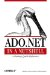# 7.11 Performing Aggregate Calculations

 The Compute( ) method computes the result of an aggregate query on the rows in the table that meet the filter criteria. Here's an example that illustrates this: ` DataTable dt = new Table(); dt.Columns.Add("OrderId", typeof(System.Int32)); dt.Columns.Add("OrderAmount", typeof(System.Decimal)); // ... add some rows // computes the sum of order amounts for all orders with Id less than 10 Decimal totalOrderAmount = dt.Compute("SUM(OrderAmount)", "OrderId<10") `